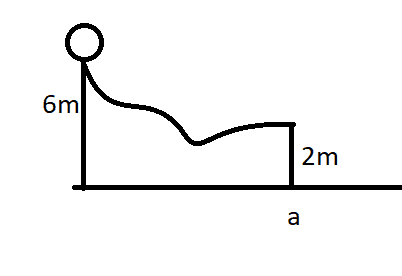# Smooth rolling ball rolls down hill, how far can it fly?

Homework Statement:
A solid ball rolls smoothly from rest (At 6m) until it leaves the ramp (at 2m). How far horizontally from point 'a' does the ball hit the ground?
Hint: Smooth rolling means it does not slide.
Relevant Equations:
v = ωr
a = αr
##K = \frac {1}{2}Iω^2 + \frac {1}{2}mv^2##Figure of the problem.

There are many exercises like these, and I've read the whole chapter and I got no clue where to start here.
Kinetic energy from gravity is: E = mgh = 39.3m
I could try change v to ωr in the K equation but it will leave me nowhere because I don't have the mass nor the radius of the ball.
I'm obviously looking for the velocity at point v, assuming it has no vertical component (altho that's not specified either), but I have no way of getting it.

Anyone has a clue what to do here?

hutchphd
Homework Helper
Do algebra. In the end what is requested is a distance......so write it down using r and v and m. Stuff will cancel.

•PeroK
Homework Helper
Gold Member
2020 Award
I could try change v to ωr in the K equation but it will leave me nowhere because I don't have the mass nor the radius of the ball.

Given that gravity acts equally on all masses, the mass in a gravitational problem such as this is never relevant.

Not quite so obvious is that for rolling without slipping, only the geometry of an object is relevant. All solid spheres roll downhill under gravity at the same rate; all hollow spheres at the same rate; all solid cylinders at the same rate etc.

As @hutchphd suggests, if you do the algebra you'll see this for yourself - in this case for solid spheres.

••